Updated by Matt Gordon on Jan 22, 2019
REPORTMatt Gordon
Owner
7 items   1 followers   0 votes   20 views

# Scientific Calculator for Chemistry Student

The chemistry student uses a Scientific Calculator. Because in simple calculator limited function are available. But in scientific calculator, multiple functions are available like trigonometric functions, logarithms, roots, powers, reciprocals, polar/rectangular functions, etc. In a market different type of scientific calculator is available. So here I provide a list of Scientific Calculator.

1

## Casio fx-115ES PLUS Calculator for Chemistry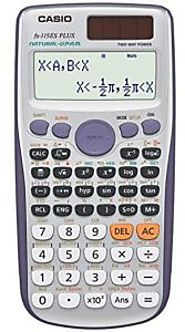The Casio fx-115ES PLUS Calculator is work to more than 280 functions. In this advanced and new feature are available. this is simple to use. That is 40 metric conversions and improved math functionality.

2

## Helect H1002 Scientific Calculator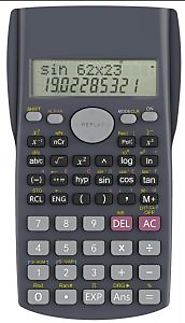The Helect H1002 Scientific Calculator has a two-line display. This Scientific Calculator is used by professional. It has a lifetime warranty. That price is low. It have a multipal function are their.

3

## Texas TI-30Xa Scientific Calculator for Chemistry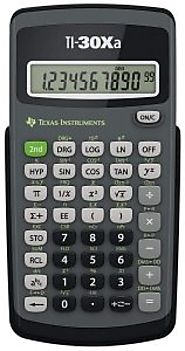In this Texas TI-30Xa Scientific Calculator have ten digits display and one line. That has slide case to keep the calculator safe. This calculator is perfect for high school students.

4

## Casio fx-300MS Scientific Calculator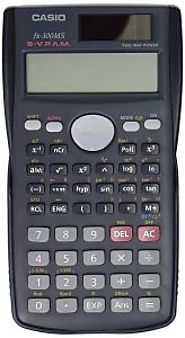The Casio fx-300MS Scientific Calculator has a multi-relay function.It is 18 levels of parentheses. It also has the solar batteries that remove the need of replacing batteries. In 229 built-in functions are available.

5

## HP Scientific Calculator for Chemistry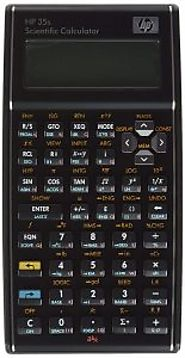In HP Scientific Calculator for Chemistry have two lines display and an LCD screen. It is used with different subjects like Physics, Chemistry, Mathematics, etc. And it has one year warranty.That have premium quality.

6

## Sharp EL-531G BBW Scientific Calculator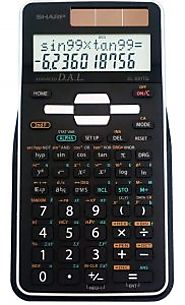The Sharp EL-531G BBW Scientific Calculator has 273 functions to help with complex calculations. It has two different mathod like simple Calculator and a scientific calculator. In this calculator 3D light reflecting cover.

7

## HP F2240AA 300s Scientific Calculator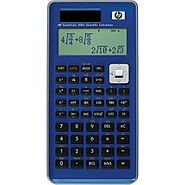The HP F2240AA 300s Scientific Calculator is used with different subjects such as algebra, calculus, physics, chemistry, earth sciences etc. It is a slide-on cover.